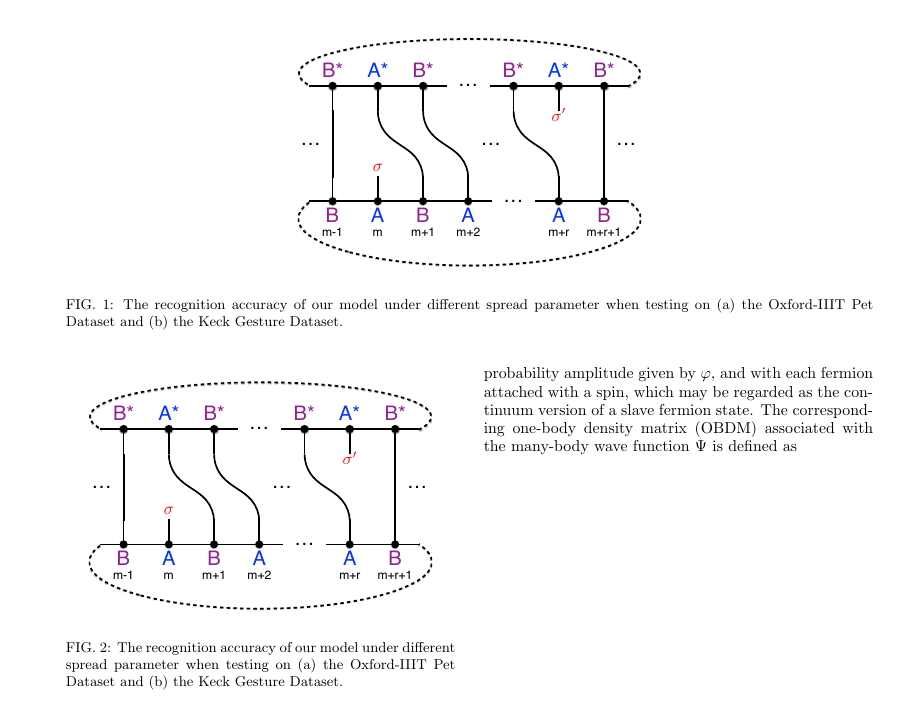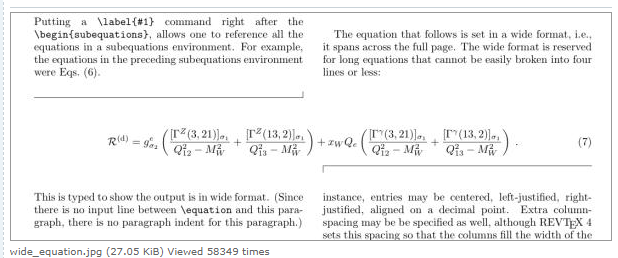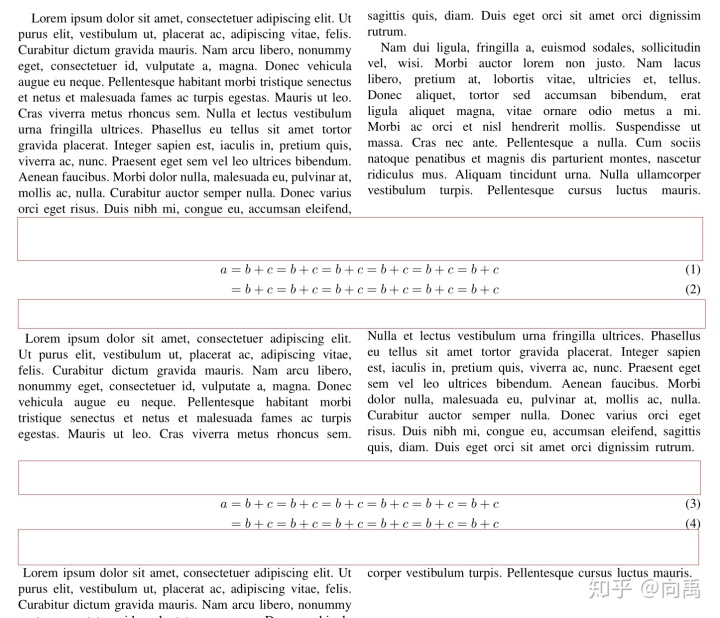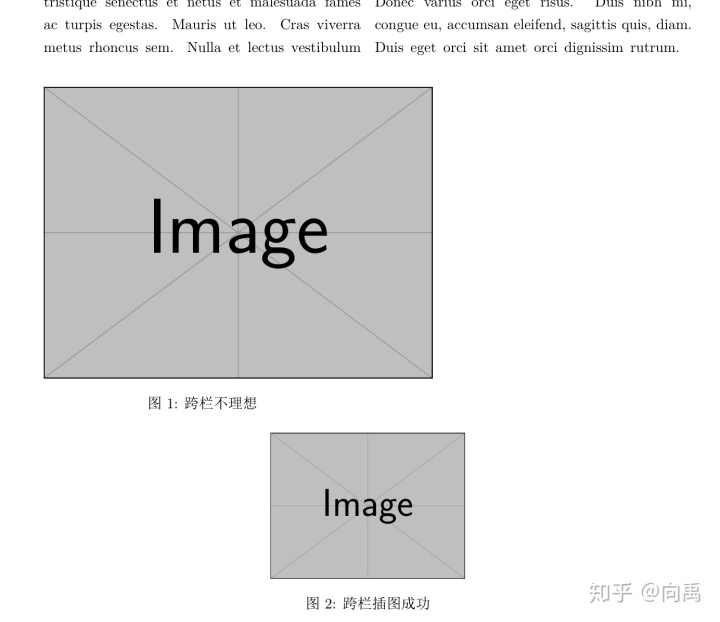• latex双栏模板
千次阅读
2020-12-01 18:06:27

# latex双栏模板中使用 通栏的公式或者图

## 通栏的图

在原来插图的环境中的 figure后加*；具体如下

\begin{figure*}[ht]
\label{fig1}
\centering
\includegraphics[width = 3in]{Fig1.pdf}
\caption{The recognition accuracy of our model under different spread parameter when testing on (a) the Oxford-IIIT Pet Dataset and (b) the Keck Gesture Dataset.}
\end{figure*}

\begin{figure}[ht]
\label{fig1}
\centering
\includegraphics[width = 3in]{Fig1.pdf}
\caption{The recognition accuracy of our model under different spread parameter when testing on (a) the Oxford-IIIT Pet Dataset and (b) the Keck Gesture Dataset.}
\end{figure}


效果图## 通栏公式

在公式放入widetext环境中

\begin{widetext}
\end{widetext}

更多相关内容
• Elsevier 双栏模板下的长公式 \documentclass[5p,preprint,12pt]{elsarticle} \usepackage{amsmath} \usepackage{...（1）LaTeX双栏模板插入通栏公式（跨栏插图） （2）cuted – Mixing onecolumn and twocolumn modes

Elsevier 双栏模板下的长公式

\documentclass[5p,preprint,12pt]{elsarticle}
\usepackage{amsmath}
\usepackage{mathtools, cuted}
\usepackage[english]{babel}
\usepackage{lipsum}
\begin{document}

\lipsum[1-2]
\begin{strip}
\begin{equation}
\sum_{q_\mathrm{tot}=0}^{Q_A+Q_B-2}\sum_{q_A=0}^{q_\mathrm{tot}}{\textstyle\binom{Q_A-1}{q_A}\binom{Q_B-1}{q_B}}x^{q_\mathrm{tot}},
\end{equation}
\end{strip}
\lipsum[1-3]

\end{document}

### 原文：long equation across whole page in two-column document

\documentclass{article}
\usepackage[margin=3cm]{geometry}
\usepackage{lipsum,amsmath,multicol}
\begin{document}

\begin{multicols}{2}
\lipsum[1-3]
\end{multicols}
\par\noindent\rule{\dimexpr(0.5\textwidth-0.5\columnsep-0.4pt)}{0.4pt}%
\rule{0.4pt}{6pt}

\begin{equation}
\mathcal{R}^{(\text{d})}=
g_{\sigma_2}^e
\left(
\frac{[\Gamma^Z(3,21)]_{\sigma_1}}{Q_{12}^2-M_W^2}
+\frac{[\Gamma^Z(13,2)]_{\sigma_1}}{Q_{13}^2-M_W^2}
\right)
+ x_WQ_e
\left(
\frac{[\Gamma^\gamma(3,21)]_{\sigma_1}}{Q_{12}^2-M_W^2}
+\frac{[\Gamma^\gamma(13,2)]_{\sigma_1}}{Q_{13}^2-M_W^2}
\right)\;. \label{eq:wideeq}
\end{equation}

\vspace{\belowdisplayskip}\hfill\rule[-6pt]{0.4pt}{6.4pt}%
\rule{\dimexpr(0.5\textwidth-0.5\columnsep-1pt)}{0.4pt}
\begin{multicols}{2}
\lipsum[4-5]
\end{multicols}

\end{document}参考资料

展开全文• 论文排版为双栏，图片也是双栏，想让图片出现在当前栏的顶端或底端，需要什么代码？</p>
• ## latex论文模板双栏

千次阅读 2021-09-01 09:12:46
最近导师让每周发汇报的时候按IEEE中双栏论文的格式发，看了网上的论文格式，这个格式还挺好用的。 会议论文 期刊论文

最近导师让每周发汇报的时候按IEEE中双栏论文的格式发，看了网上的论文格式，这个格式还挺好用的。

1. 会议论文2. 期刊论文百度云链接
链接：https://pan.baidu.com/s/1LjMiyRratUvFUul6UsYBVw
提取码：3obr

展开全文• 带星号的浮动体figure和table环境可以在双栏模板中使用，例如我们以IEEEtran为例，插入通栏公式 \documentclass{IEEEtran} \usepackage{amsmath，lipsum} \begin{document} \lipsum \begin{figure*} \begin{align...

### 1. 利用带星号的浮动体环境

带星号的浮动体figure和table环境可以在双栏模板中使用，例如我们以IEEEtran为例，插入通栏公式

\documentclass{IEEEtran}
\usepackage{amsmath，lipsum}
\begin{document}
\lipsum
\begin{figure*}
\begin{align}
a&=b+c=b+c=b+c=b+c=b+c=b+c
&=b+c=b+c=b+c=b+c=b+c=b+c
\end{align}
\end{figure*}
\lipsum[1-10]
\end{document}


可以发现，这样确实实现了公式的通栏，但是这个带星号的浮动体只会出现在下一页的顶部，无法出现在当前位置，所以会使得公式的顺序紊乱，如果手动将公式提前，使得它出现在当前页的顶部，会使得公式的编号紊乱，需要手动更改计数器equation的值，这样并不是一个最好的方法.

### 2. 利用cuted包提供的strip环境

cuted包可以说是完全解决了一个这样的问题，把公式环境用strip环境包裹，可以直接在当前位置插入通栏公式

\documentclass{IEEEtran}
\usepackage{amsmath,lipsum}
\usepackage{cuted}%%stripsep-3pt
\begin{document}
\lipsum[1-2]
\begin{strip}
\begin{align}
a&=b+c=b+c=b+c=b+c=b+c=b+c
&=b+c=b+c=b+c=b+c=b+c=b+c
\end{align}
\end{strip}
\lipsum
\begin{strip}
\begin{align}
a&=b+c=b+c=b+c=b+c=b+c=b+c
&=b+c=b+c=b+c=b+c=b+c=b+c
\end{align}
\end{strip}
\lipsum[1-2]
\end{document}


效果如下不过大家发现这里的公式上下似乎出现了不小的间距，小问题，在我的代码中有一个带注释的命令stripsep，这个命令就是strip环境的前后弹性粘连(间距)，它的默认值为15pt plus 2pt minus 2pt，可以更改它的值来改变这个间距.例如这里的stripsep-3pt或者stripsep=-3pt将使得strip环境的前后间距设置为-3pt，不过我们还是建议大家将这个间距设置为弹性距离，方便在换页时候的排版，例如stripsep -3pt plus 3pt minus 2pt.这样的话，cuted包就彻底解决了双栏模板的通栏公式问题.

### 3. 双栏模板下插入跨栏的图片（表格）

对于一些强迫症患者，不仅希望公式当前位置，甚至也希望图表也在当前位置。原则上图表用figure和table环境可以使得图表在双栏模板下浮动到下一页的顶部，然后实现交叉引用即可，但是如果借助cuted包也可以实现将图表放在当前位置。在这里首先要注意的问题是，strip环境其实相当于是一个盒子，浮动体是不能放在限制浮动的盒子里面的，因此第一个想法是用float包，然后begin{figure}[H]放在strip环境中取消figure环境的浮动，但是这么做之后得到的效果并不理想，甚至也可以说是失败。一番尝试以后，我找到了另一个方法，就是加载caption宏包，不用figure环境而直接使用captionof命令。下面的mwe经过调试大家就知道了

\documentclass[twocolumn]{ctexart}
\usepackage{lipsum,mwe,cuted}
\usepackage{float}%%%%提供浮动体的[H]选项，进而取消浮动
\usepackage{caption}%%提供captionof命令
\stripsep-2pt
\begin{document}
\lipsum
\begin{strip}
\begin{figure}[H]
\centering
\includegraphics[width=0.6textwidth]{example-image}
\caption{跨栏不理想}
\end{figure}
\end{strip}

\begin{strip}
\centeringincludegraphics[width=0.3textwidth]{example-image}
\captionof{figure}{跨栏插图成功}
\end{strip}

\lipsum[1-2]
\end{document}https://blog.csdn.net/weixin_39942033/article/details/110606149

展开全文• 模板语句 \documentclass[Afour,sageh,times]{sagej} 更改为 \documentclass[Royal,sageh,times]{sagej} 即可实现双栏转换为单栏
• 问题描述： 图片标题无法居中，始终靠左。 在\figure下用\centering 不行，宏包\usepackage[justification=centering]{caption} 也不管用），宏包\usepackage{caption2}不行，\begin{table}后面的 [pos=hbt!...css html css3
• Elsevier-template-d.doc是双栏模板，Elsevier-template-s.doc是单栏模板
• \usepackage{wrapfig} \begin{wrapfigure}{l}[0em]{0.15\textwidth}%靠文字内容的左侧 \includegraphics[width=0.15\textwidth]{figure/XXX.png} \end{wrapfigure} \textbf {XXX} received the B.S....
• 1.问题：（使用latex版本的双栏模板时，图片名称不显示） 在网上下载了一个双栏的latex模板。 正常情况下，使用latex插入图片时，应该如下图所示，显示出图片+图片名称。 但我使用以下代码插入图片，出现问题...
• ## latex双栏文章分页

千次阅读 2019-08-02 18:13:37
标题：latex 双栏文章分页 方案： 因为是双栏latex文章，所以如果输入： \newpage ，只能做到单栏分页（即后面的内容会移到右边一栏，而不是下一页）。 如果输入 \pagebreak ，更加得不到预期分页结果。...
• 关于的入门，可以参考：《LaTeX环境配置》，和《LaTeX入门(一)》当然，我不打算继续从 入门(二)开始写起，关于这个新的专题：学习指南，将会一直写下去，与 R 语言系列一样不断地更新，同时，我希望，尽可能地解释...
• LaTeX表格排版的技巧，表格占满单栏布局宽度，表格占满单栏布局中两栏宽度，或者自定义表格宽度(无论是单栏还是双栏)
• 问题描述：在投稿论文的过程中，使用elsevier的cas-dc模板，单图片可以很好的显示，但是遇到跨栏的图片\caption不会自动左对齐。 如下所示，跨栏图片的标题显示不正常。 \caption出现在中间位置，这并不是...
• 问题如下图所示，图片标题无法居中： 贴上代码：（用了\centering 也不管用） \begin{figure}[htbp] \centering {\includegraphics[scale=.75]{figs/Fig1.pdf}} \caption{The test figure.} \label{fig1} ...
• \twocolumn[ \begin{@twocolumnfalse} \section*{\centering{Supplementary Material for \\ \emph{Learning in the Frequency Domain}}} \end{@twocolumnfalse} ] 效果如下：
• Latex 英文论文双排模板 可以直接在上面修改然后生成
• 论文返修过程中为了方便审稿人审阅，需要为返回稿...\switchlinenumbers %编号在左的左侧和右的右侧 \end{document} latex中实现 page 当前页 of 总页数 效果 \usepackage{fancyhdr} \usepackage{lastpage...
• 作　者：marsggbo出　处：https://www.cnblogs.com/marsggbo科技文档排版时，有时表格可能一页都装不下，或者双栏模板单栏不够长，如果用原始的table包的话很可能就会溢出了，所以很自然的一个想法是让表格自动换页...
• 代码】记录|Latex双栏排版插入图片后图片太大的问题一种解决方案。图片
• latex论文版本中，经常会有双栏格式下对摘要进行单栏设置，查阅无数博客始终无效，两天摸索下终于完工！真是不容易啊！！本文主要包括下面内容： 摘要单栏设置 摘要单栏下脚注消失问题 如何添加脚注 脚注无序号+...
• 在table or figure里多加一个星号就实现了完美的单栏表格或图片排版在双栏的文章里。 表格横跨两栏代码 \begin{table*} … \end{table*} 图形横跨两栏代码 \begin{figure*} … \end{figure*}
• 我的一门光纤课的大作业，利用latex编写的，双栏的，摘要，reference齐全，包括图片的插入等都有。我自认为是很好的latex中文论文的模板。没有不必要的宏包和乱七八糟的注释。
• 1. 照片双栏格式插入 \begin{figure} \includegraphics[width=\linewidth]{qian.jpg} \caption{Example of a figure caption.} \label{qian.jpg} \end{figure} 2. 照片单栏格式插入 \begin{figure*} \...ieee论文
• ## LaTeX在双栏模式下插入跨栏图表

万次阅读 多人点赞 2016-06-10 19:50:11
LaTeX中插入eps图片的命令是： \begin{figure} \centering \includegraphics[width=8cm]{picture.eps} ...在双栏编辑模式下，图片只能在一栏中显示，而且如果图片的宽度超过单栏文本宽度，则只能显示其中一部模式 插入...